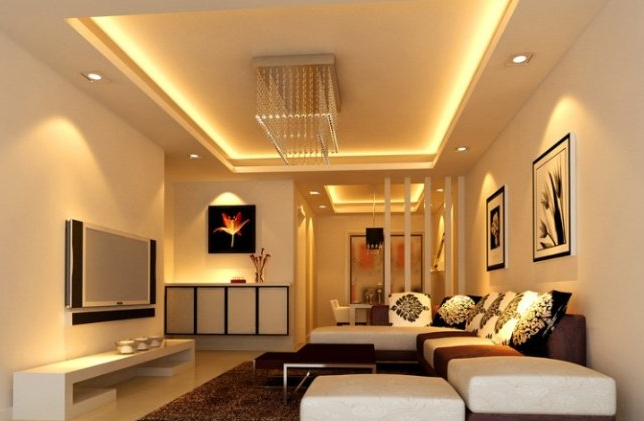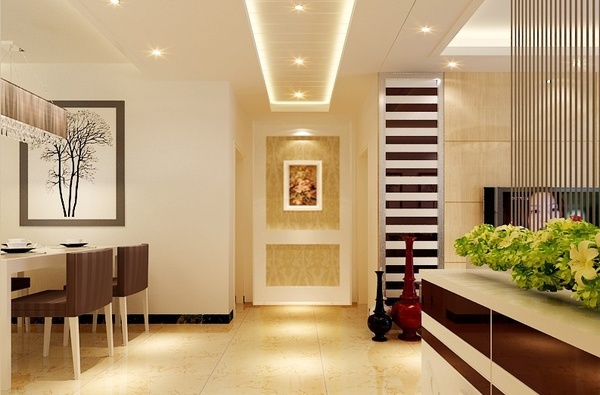|

# 吊顶 的装修误区

我们家中的装修是有很多讲究的，像是天花板的装修，也就是吊灯。它的装修的工程算是上家居装修的一个重要的部分了。一些没有装修经验的业主在做的时候，往往会走进误区。下面，我们就来了解下关于吊顶装修的误区。一起来了解下。客厅吊顶图一

Part1：安装优质吊顶 首先避免选材误区

选材是家居装修的第一步，很多人基本看不到吊顶材料的检测报告，因此只能通过自己看来选择吊顶，所以消费者还得自己动手才能挑到好材料，那就必须避免一下四个误区。

误区1：莫让价格蒙蔽双眼

吊顶有金属吊顶和石膏吊顶之分，一般客厅采用石膏吊顶，而卫浴厨房等使用金属吊顶。尽管是同种吊顶，价格也有差别，有些人以为吊顶只是一小部分的装修，购买平价材料；有的人则看重贵价材料，认为越贵越好。这些盲目追求价格的购买方式，会导致错误选材。

支招：对于金属吊顶来说，板材因铝的纯度和表面工艺不一样，价格差别比较大。最好的扣板是进口LG覆膜板，无色差，30年不变色。最差的是喷涂板，容易沾油烟，有毒性、易变色。所以广大消费者在注重价格之余要注意看清材质。

误区2：金属吊顶都环保

不要以为只有会释放甲醛的材料才会存在环保问题，许多人以为金属比较环保，对人体的害处不大，其实这也是吊顶选购时候的一些常见误区。

支招：优质金属板却是比较环保，但是劣质金属板含重金属，对人体有辐射。而且含铁量高容易生锈，劣质覆膜易滋生细菌，LG覆膜洁净抗菌。走道吊顶图示二

误区3：只关心板材厚度

在挑选金属吊顶的时候，消费者都希望挑选质量较好的材料。有些人就盲目地以为，越厚的板质量越好。这是吊顶板材选购的常见误区之一，板材的厚度并不代表质量，反而太厚的板会影响吊顶的外观和耐用度。

支招：判断板材质量的关键是材质，铝的纯度，优质铝扣板轻，弹性好。劣质铝扣板铁、重金属含量高，板材重且容易折断。所以，并不是越厚就越好的。家居吊顶一般使用的是厚度有0.55毫米、0.6毫米、0.7毫米等规格。

误区4：板材越大越好

一些屋主认为，买板材的时候，挑选大一点的，这样用量少，能够省下不少的开销，但是结果安装的效果不好，没能做出吊顶应有的效果。

支招：吊顶扣板并非越大越好，只要达到国家标准就好，市场上的铝扣板宽度一般在10-15厘米之间。根据空间的实际情况，挑选合适大小的板材，才是精明的消费者。

Part2：莫为追求品位 巧妙防止施工误区

安装优质吊顶，不仅材料优质，而且施工更是关键，我们千万不能让施工误区让我们的吊顶前功尽弃。所以，施工误区不可小觑，下面我们就来认识一下吊顶安装的四大施工误区。

误区1：过于追求个性

有些人安装吊顶时，会设计凹凸不平的造型，认为凹凸不平的造型会突显主人个性，或使用玻璃、镜子等材料，这就给平时的清洁带来了困难，使吊顶成为了藏污纳垢之所，从而污染室内空气环境。在风沙较大、灰尘较多的北方地区尤其如此。

支招：建议消费者根据家居的实际情况安装自家的吊顶。客厅和卧室的天花，尽量使用简单线条的设计装修；卫浴和厨房建议使用普通的铝扣吊顶，玻璃等材料尽量少用，这样可避免吊顶成为藏污纳垢的地方。

误区2：吊顶无须设置维修孔

吊顶能够使家居空间更加美观，许多消费者在追求美观的同时，忽略了日后的维护。在安装吊顶时，不给吊顶留下维修口，日后吊顶维修、电线检查等工作就变得不容易了。

支招：设置检修孔可能会碍于美观，但是检修口不能缺少，因为吊顶里面可能布满了电线，以后要检修就根本找不到可以探进去的位置，所有的地方都是封死的，就得将吊顶完全揭开，这样真是得不偿失。面积小的吊顶一般保留一个500x600mm的维修口即可。

误区3：吊顶安装不须太费神

许多消费者认为，吊顶只是装修其中一个小部分，面积较小，不须花太对心思去做。最后由于安装不够细致，导致吊顶不牢固，往往为家居安全种下祸根。

支招：吊顶不牢固是祸根，吊顶安装不牢固的话，就会有垮塌的危险。所以，在安装时，一定要将吊顶龙骨、板材等安装牢固，千万不要种下祸根。

屋企4：盲目安装吊顶

不少消费者认为家庭装修要吊顶才显得够档次，实际上，由于现在的商品房层高上不如以前，通常只有2.6米左右。如果这样一个相对狭小的空间全部安装吊顶，在视觉上会使人感到紧张、压抑，继而引发一些生理上的反应，如头晕、恶心等。

支招：并非所有空间都适合安装吊顶的，而且吊顶的高度也需要计算好。如果房屋高度只有2.6-2.8米，最好选择在天花四周做简单的吊顶，不宜做得太大面积，一般吊顶的高度在2.3-2.6米左右。

Part3：保障吊顶施工 小心莫入验收误区

吊顶工程范围较广，但无论哪一种类型，合理的设计、优质的材料、规范的施工工艺，是保证质量与安全的重要因素。此外，验收工作也必不可少，以下是验收的四大误区，了解误区注意避免进入验收误区。

误区1：验收不检查清洁度

也许有些人会认为，吊顶不会有灰尘，不需要清洁检查，但如果吊顶是迭级凹凸的造型，就很容易藏污纳垢。

支招：吊顶容易藏进灰尘等，在装修验收时候，对吊顶的清洁度也需要进行检查。一般需要检查吊顶的表面清洁度，吊顶空隙位置是否有杂物，或者施工留下的废料等。

误区2：扣板吊顶反光度高才好

在检查吊顶安装时，有些业主会纠结吊顶板材表面光滑度不够，影响整体的美观度，希望师傅再进行打磨，其实，这种想法是吊顶验收时的一大误区。

支招：安装的吊顶表面需要无凹凸不平，比较光滑即可，并不需要过于光滑。过于光滑的表面反而导致空间反光度增加，易造成家居光污染。

误区3：吊顶越平整越好

验收时通常存在这样的误区，吊顶天花必须安装平整。因此，业主们在验收时对天花的平整度的检查非常严格，认为天花平整度的要求与地面墙面平整度要求相同，其实这种想法是不正确的。

支招：吊顶平稳是好，但是也并非越平整越好的，需要考虑到吊顶龙骨日后下垂问题，所以检查的时候，一般吊顶中心应按短边起拱1/200符合标准。

`声明：本文由入驻焦点开放平台的作者撰写，除焦点官方账号外，观点仅代表作者本人，不代表焦点立场错误信息举报电话： 400-099-0099，邮箱：jubao@vip.sohu.com，或点此进行意见反馈，或点此进行举报投诉。`A B C D E F G H J K L M N P Q R S T W X Y Z
A - B - C - D - E
• A
• 鞍山
• 安庆
• 安阳
• 安顺
• 安康
• 澳门
• B
• 北京
• 保定
• 包头
• 巴彦淖尔
• 本溪
• 蚌埠
• 亳州
• 滨州
• 北海
• 百色
• 巴中
• 毕节
• 保山
• 宝鸡
• 白银
• 巴州
• C
• 承德
• 沧州
• 长治
• 赤峰
• 朝阳
• 长春
• 常州
• 滁州
• 池州
• 长沙
• 常德
• 郴州
• 潮州
• 崇左
• 重庆
• 成都
• 楚雄
• 昌都
• 慈溪
• 常熟
• D
• 大同
• 大连
• 丹东
• 大庆
• 东营
• 德州
• 东莞
• 德阳
• 达州
• 大理
• 德宏
• 定西
• 儋州
• 东平
• E
• 鄂尔多斯
• 鄂州
• 恩施
F - G - H - I - J
• F
• 抚顺
• 阜新
• 阜阳
• 福州
• 抚州
• 佛山
• 防城港
• G
• 赣州
• 广州
• 桂林
• 贵港
• 广元
• 广安
• 贵阳
• 固原
• H
• 邯郸
• 衡水
• 呼和浩特
• 呼伦贝尔
• 葫芦岛
• 哈尔滨
• 黑河
• 淮安
• 杭州
• 湖州
• 合肥
• 淮南
• 淮北
• 黄山
• 菏泽
• 鹤壁
• 黄石
• 黄冈
• 衡阳
• 怀化
• 惠州
• 河源
• 贺州
• 河池
• 海口
• 红河
• 汉中
• 海东
• I
• J
• 晋中
• 锦州
• 吉林
• 鸡西
• 佳木斯
• 嘉兴
• 金华
• 景德镇
• 九江
• 吉安
• 济南
• 济宁
• 焦作
• 荆门
• 荆州
• 江门
• 揭阳
• 金昌
• 酒泉
• 嘉峪关
K - L - M - N - P
• K
• 开封
• 昆明
• 昆山
• L
• 廊坊
• 临汾
• 辽阳
• 连云港
• 丽水
• 六安
• 龙岩
• 莱芜
• 临沂
• 聊城
• 洛阳
• 漯河
• 娄底
• 柳州
• 来宾
• 泸州
• 乐山
• 六盘水
• 丽江
• 临沧
• 拉萨
• 林芝
• 兰州
• 陇南
• M
• 牡丹江
• 马鞍山
• 茂名
• 梅州
• 绵阳
• 眉山
• N
• 南京
• 南通
• 宁波
• 南平
• 宁德
• 南昌
• 南阳
• 南宁
• 内江
• 南充
• P
• 盘锦
• 莆田
• 平顶山
• 濮阳
• 攀枝花
• 普洱
• 平凉
Q - R - S - T - W
• Q
• 秦皇岛
• 齐齐哈尔
• 衢州
• 泉州
• 青岛
• 清远
• 钦州
• 黔南
• 曲靖
• 庆阳
• R
• 日照
• 日喀则
• S
• 石家庄
• 沈阳
• 双鸭山
• 绥化
• 上海
• 苏州
• 宿迁
• 绍兴
• 宿州
• 三明
• 上饶
• 三门峡
• 商丘
• 十堰
• 随州
• 邵阳
• 韶关
• 深圳
• 汕头
• 汕尾
• 三亚
• 三沙
• 遂宁
• 山南
• 商洛
• 石嘴山
• T
• 天津
• 唐山
• 太原
• 通辽
• 铁岭
• 泰州
• 台州
• 铜陵
• 泰安
• 铜仁
• 铜川
• 天水
• 天门
• W
• 乌海
• 乌兰察布
• 无锡
• 温州
• 芜湖
• 潍坊
• 威海
• 武汉
• 梧州
• 渭南
• 武威
• 吴忠
• 乌鲁木齐
X - Y - Z
• X
• 邢台
• 徐州
• 宣城
• 厦门
• 新乡
• 许昌
• 信阳
• 襄阳
• 孝感
• 咸宁
• 湘潭
• 湘西
• 西双版纳
• 西安
• 咸阳
• 西宁
• 仙桃
• 西昌
• Y
• 运城
• 营口
• 盐城
• 扬州
• 鹰潭
• 宜春
• 烟台
• 宜昌
• 岳阳
• 益阳
• 永州
• 阳江
• 云浮
• 玉林
• 宜宾
• 雅安
• 玉溪
• 延安
• 榆林
• 银川
• Z
• 张家口
• 镇江
• 舟山
• 漳州
• 淄博
• 枣庄
• 郑州
• 周口
• 驻马店
• 株洲
• 张家界
• 珠海
• 湛江
• 肇庆
• 中山
• 自贡
• 资阳
• 遵义
• 昭通
• 张掖
• 中卫

1室1厅1厨1卫1阳台

1
2
3
4
5

0
1
2

1

1

0
1
2
3报名成功，资料已提交审核A B C D E F G H J K L M N P Q R S T W X Y Z
A - B - C - D - E
• A
• 鞍山
• 安庆
• 安阳
• 安顺
• 安康
• 澳门
• B
• 北京
• 保定
• 包头
• 巴彦淖尔
• 本溪
• 蚌埠
• 亳州
• 滨州
• 北海
• 百色
• 巴中
• 毕节
• 保山
• 宝鸡
• 白银
• 巴州
• C
• 承德
• 沧州
• 长治
• 赤峰
• 朝阳
• 长春
• 常州
• 滁州
• 池州
• 长沙
• 常德
• 郴州
• 潮州
• 崇左
• 重庆
• 成都
• 楚雄
• 昌都
• 慈溪
• 常熟
• D
• 大同
• 大连
• 丹东
• 大庆
• 东营
• 德州
• 东莞
• 德阳
• 达州
• 大理
• 德宏
• 定西
• 儋州
• 东平
• E
• 鄂尔多斯
• 鄂州
• 恩施
F - G - H - I - J
• F
• 抚顺
• 阜新
• 阜阳
• 福州
• 抚州
• 佛山
• 防城港
• G
• 赣州
• 广州
• 桂林
• 贵港
• 广元
• 广安
• 贵阳
• 固原
• H
• 邯郸
• 衡水
• 呼和浩特
• 呼伦贝尔
• 葫芦岛
• 哈尔滨
• 黑河
• 淮安
• 杭州
• 湖州
• 合肥
• 淮南
• 淮北
• 黄山
• 菏泽
• 鹤壁
• 黄石
• 黄冈
• 衡阳
• 怀化
• 惠州
• 河源
• 贺州
• 河池
• 海口
• 红河
• 汉中
• 海东
• I
• J
• 晋中
• 锦州
• 吉林
• 鸡西
• 佳木斯
• 嘉兴
• 金华
• 景德镇
• 九江
• 吉安
• 济南
• 济宁
• 焦作
• 荆门
• 荆州
• 江门
• 揭阳
• 金昌
• 酒泉
• 嘉峪关
K - L - M - N - P
• K
• 开封
• 昆明
• 昆山
• L
• 廊坊
• 临汾
• 辽阳
• 连云港
• 丽水
• 六安
• 龙岩
• 莱芜
• 临沂
• 聊城
• 洛阳
• 漯河
• 娄底
• 柳州
• 来宾
• 泸州
• 乐山
• 六盘水
• 丽江
• 临沧
• 拉萨
• 林芝
• 兰州
• 陇南
• M
• 牡丹江
• 马鞍山
• 茂名
• 梅州
• 绵阳
• 眉山
• N
• 南京
• 南通
• 宁波
• 南平
• 宁德
• 南昌
• 南阳
• 南宁
• 内江
• 南充
• P
• 盘锦
• 莆田
• 平顶山
• 濮阳
• 攀枝花
• 普洱
• 平凉
Q - R - S - T - W
• Q
• 秦皇岛
• 齐齐哈尔
• 衢州
• 泉州
• 青岛
• 清远
• 钦州
• 黔南
• 曲靖
• 庆阳
• R
• 日照
• 日喀则
• S
• 石家庄
• 沈阳
• 双鸭山
• 绥化
• 上海
• 苏州
• 宿迁
• 绍兴
• 宿州
• 三明
• 上饶
• 三门峡
• 商丘
• 十堰
• 随州
• 邵阳
• 韶关
• 深圳
• 汕头
• 汕尾
• 三亚
• 三沙
• 遂宁
• 山南
• 商洛
• 石嘴山
• T
• 天津
• 唐山
• 太原
• 通辽
• 铁岭
• 泰州
• 台州
• 铜陵
• 泰安
• 铜仁
• 铜川
• 天水
• 天门
• W
• 乌海
• 乌兰察布
• 无锡
• 温州
• 芜湖
• 潍坊
• 威海
• 武汉
• 梧州
• 渭南
• 武威
• 吴忠
• 乌鲁木齐
X - Y - Z
• X
• 邢台
• 徐州
• 宣城
• 厦门
• 新乡
• 许昌
• 信阳
• 襄阳
• 孝感
• 咸宁
• 湘潭
• 湘西
• 西双版纳
• 西安
• 咸阳
• 西宁
• 仙桃
• 西昌
• Y
• 运城
• 营口
• 盐城
• 扬州
• 鹰潭
• 宜春
• 烟台
• 宜昌
• 岳阳
• 益阳
• 永州
• 阳江
• 云浮
• 玉林
• 宜宾
• 雅安
• 玉溪
• 延安
• 榆林
• 银川
• Z
• 张家口
• 镇江
• 舟山
• 漳州
• 淄博
• 枣庄
• 郑州
• 周口
• 驻马店
• 株洲
• 张家界
• 珠海
• 湛江
• 肇庆
• 中山
• 自贡
• 资阳
• 遵义
• 昭通
• 张掖
• 中卫• 手机• 分享
• 设计
免费设计
• 计算器
装修计算器
• 入驻
合作入驻
• 联系
联系我们
• 置顶
返回顶部